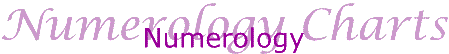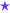## The Meaning of Numbers - Numerology

Most people are aware of the "quantity" value of numbers but there is also a "quality" associated with numbers as well. Developed by Pythagoras over 2,500 years ago, the meaning of numbers can be a useful aid in self-development and understanding character traits.

### Here are some of the basics on how Numerology works

The numbers 1 to 9 are the building blocks of numerology and therefore all other numbers are reduced to a single digit by adding each digit together until the number is reduced down to just one digit.

For example the number 68 is considered as two digits 6 and 8 which when added together is equal to 14. If we do the same to 14 (1 and 4) then these when added together equal 5. This gives a reduction of the number "68" to it's core value of "5".

#### Ruling Number

The numbers making up a person's date of birth is one example of the use of numerology and these can be used to give an indication of the qualitites that a person was born with.

For example:

A birth date of December 23rd, 1998 [24/12/1993] produces a Ruling Number deermined by adding together each number in the date of birth i.e.

2 + 4 + 1 + 2 + 1 + 9 + 9 + 3 = 31

The 31 is further reduced by adding 1 + 3 = 4. So the ruling number of someone born on the 23rd December 1998 is a 4.

#### Names

Names can also be translated into numbers. This is done by converting each letter into a number from 1 to 9 according to the following table. So A = 1, B = 2 and so on.

 1 2 3 4 5 6 7 8 9 A B C D E F G H I J K L M N O P Q R S T U V W X Y Z

As an example, the name John is made up of the numbers 1 and 6 and 8 and 5. John has the values of each of these numbers as part of his personality make up. Adding all of the numbers together gives a total for the name as well 1 + 6 + 8 + 5 = 20. Numbers greater than 9 are reduced to a single digit by adding them together. In this case 2 + 0 = 2.HomePalmistryNumerology